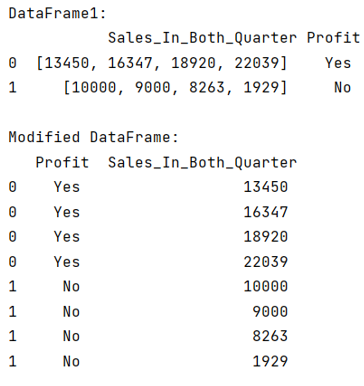# Python Pandas: Flatten a list of dataframe

Learn, how to flatten a list of pandas dataframe?
Submitted by Pranit Sharma, on August 05, 2022

Pandas is a special tool that allows us to perform complex manipulations of data effectively and efficiently. Inside pandas, we mostly deal with a dataset in the form of DataFrame. DataFrames are 2-dimensional data structures in pandas. DataFrames consist of rows, columns, and data.

In this article, we are going to learn how to flatten a DataFrame to a list, when we convert or change the data format to a narrow format, it is called flattening. The advantage of the flattened list is increasing the computing speed and helps in better understanding of data and effective data analysis.

A list is a collection of the heterogeneous type of elements which means the data inside a list can be of any type. A list in python is just like an array where the first element has an index of 1 and the last element has an index n-1 where n is the length of the list.

To flatten a DataFrame into a list, we will first create a DataFrame with a column having a list of multiple elements. We will first loop over this column and then we will again loop over each list of this column to store every element of this list into a separate variable. We will also access the index value of column values which will help us to identify the data.

Finally, we will create another dataframe with all the gained values.

Let us understand with the help of an example,

## Python code to flatten a list of pandas dataframe

```# Importing pandas package
import pandas as pd

# Creating a dictionary
d = {
'Sales_In_Both_Quarter':[[13450,16347,18920,22039],[10000,9000,8263,1929]],
'Profit':['Yes','No']
}

# Creating a DataFrame
df = pd.DataFrame(d)

# Display DataFrame
print("DataFrame1:\n",df,"\n")

# Accessing values and flattening the list
flatdata = pd.DataFrame([(index, value) for (index, values)
in df['Sales_In_Both_Quarter'].iteritems() for value in values],
columns=['index', 'Sales_In_Both_Quarter']).set_index('index')

# merging with previous dataframe
df = df.drop('Sales_In_Both_Quarter', axis=1).join(flatdata)

# Display modified DataFrame
print("Modified DataFrame:\n",df)
```

Output:Languages: » C » C++ » C++ STL » Java » Data Structure » C#.Net » Android » Kotlin » SQL
Web Technologies: » PHP » Python » JavaScript » CSS » Ajax » Node.js » Web programming/HTML
Solved programs: » C » C++ » DS » Java » C#
Aptitude que. & ans.: » C » C++ » Java » DBMS
Interview que. & ans.: » C » Embedded C » Java » SEO » HR
CS Subjects: » CS Basics » O.S. » Networks » DBMS » Embedded Systems » Cloud Computing
» Machine learning » CS Organizations » Linux » DOS
More: » Articles » Puzzles » News/Updates